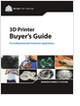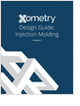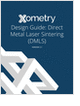Design HomeGearsIntroductionHistoryNomenclatureCompound TrainEpicyclic Gear TrainRatio CalculationExampleAutomobile DifferentialResourcesBibliography
Selecting the Right 3D Printer

Discover how to choose the right 3D printer for your needs and the key performance attributes to consider.

CNC Machining Design Guide

Injection Molding Design Guide

Guide for high quality and cost-effective plastic injection molding.

Metal 3D Printing Design Guide

Direct Metal Laser Sintering (DMLS) 3D printing for parts with reduced cost and little waste.

more free publicationsEpicyclic Train Overall Ratio CalculationWe present here a simple, methodical procedure for determining the final gear ratio of an epicyclic gear train. This method is extremely procedural, which is appropriate since use of intuition is often quite futile when applied to complex gear trains.

We can use the following superposition concept for determining rotational speeds of the gears:

Rotationplanet gear = Rotationarm + Rotationplanet gear relative to arm

In the following illustration and analysis, the sun gear has twice the diameter of the planet gear.We can analyze this non-intuitive configuration with the following table. For this discussion, a positive turn is defined to be counterclockwise.

 Action Arm Turns Planet Turns Sun Turns With all moving parts locked, the entire assembly is given one positive turn (CCW). +1 +1 +1 With the arm fixed, the sun is given one negative turn (CW). 0 (NSUN/NPLANET)=+2 -1 Add the above two values to obtain the RESULTANT TURNS. +1 +3 0

To demonstrate the derivation of the above table, we will go through the procedure step-by-step.

STEP 1 All moving parts of the train are locked with respect to each other. Then, the entire assembly is turned CCW one turn (360 degrees). The obvious result of this is that each and every element of the gear train undergoes +1 turns, as tabulated below.

 Action Arm Turns Planet Turns Sun Turns With all moving parts locked, the entire assembly is given one positive turn (CCW). +1 +1 +1

STEP 2   The arm is fixed, and the sun gear is given one negative turn (CW). Since what happens is fairly simple to visualize, the results can be predicted and tabulated as shown below.

 Action Arm Turns Planet Turns Sun Turns With all moving parts locked, the entire assembly is given one positive turn (CCW). +1 +1 +1 With the arm fixed, the sun is given one negative turn (CW). 0 (NSUN/NPLANET)=+2 -1

STEP 3   For Step 3, we simply superimpose the two effects of Step 1 and Step 2. This involves adding the turns in each step so that we sum up the columns in the table.

 Action Arm Turns Planet Turns Sun Turns With all moving parts locked, the entire assembly is given one positive turn (CCW). +1 +1 +1 With the arm fixed, the sun is given one negative turn (CW). 0 (NSUN/NPLANET)=+2 -1 Add the above two values to obtain the RESULTANT TURNS. +1 +3 0

With the rotation of each element of the gear train in hand, the overall gear ratio becomes easy. If the arm is the input and the planet is the output, the overall gear ratio is 3/1 = 3.

For a more involved epicyclic gear train example, please refer to the Epicyclic Gear Train Example section.

GlossarySelecting the Right 3D Printer

Discover how to choose the right 3D printer for your needs and the key performance attributes to consider.CNC Machining Design Guide